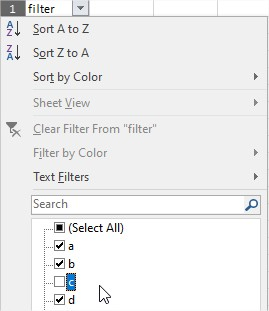# Printing df as DataTable, why aren't columns adjusting in width?

I’m trying to write a function that’ll quickly print a dataframe as a DataTable when working with pandas on any project. I can’t work out how to make column widths auto adjust:

``````import pandas as pd, numpy as np, random, string
from bokeh.models import ColumnDataSource, DataTable, TableColumn
from bokeh.plotting import show

df = pd.DataFrame(np.random.randint(0,100,size=(100, 12)), columns=[(random.randint(1,30) * random.choice(string.ascii_uppercase)) for col in range(12)])

def bokehprint(x):
show(DataTable(columns= [TableColumn(field=c, title=c, width=len(c)) for c in x.columns], fit_columns = True, sizing_mode = 'stretch_both', source=ColumnDataSource(x)))

bokehprint(df)
``````

Setting width in `TableColumn` doesn’t seem to change anything, and `fit_columns` will evenly fit columns across display width, but I’d like column widths to adapt appropriately. What am I doing wrong?

Also, there’s no way to have a drop down filter like you could in MS Excel, is there? I looked in documentation but didn’t find anything, but that’d be really nice.Crazy idea: wouldn’t it be cool for Bokeh to come with a standard df print function like this? So instead of `print(df)` any pandas user with zero understanding of Bokeh could go `from bokeh import bokehprint` and `bokehprint(df)` or similar? I reckon that might create great publicity for Bokeh and serve as a good introduction to Bokeh for prospective users.

Hi @tvkyq;

DataTables have undergone some changes recently. Make sure you’re at 2.2, and then try something like this:

``````dt = DataTable(columns=[TableColumn(field=c, title=c, width=len(c)) for c in x.columns],
autosize_mode='fit_columns',
sizing_mode='stretch_both',
source=ColumnDataSource(x))``````
1 Like

Ah, righto. I was on 2.2.3 but needed `autosize_mode = 'fit_columns'`. Thanks!

Great! And thank you for taking the time to put in a GH issue for the docs-- I agree that it’s a little light, and we’ll get that updated.

1 Like